# FFT Express VI

## LabVIEW 2018 FPGA Module Help

Edition Date: March 2018
Part Number: 371599P-01
View Product InfoLabVIEW 2016 FPGA Module HelpLabVIEW 2017 FPGA Module HelpLabVIEW 2018 FPGA Module HelpLabVIEW 2019 FPGA Module HelpLabVIEW 2020 FPGA Module Help

Owning Palette: FPGA Math & Analysis VIs and Functions

Requires: FPGA Module

Computes the Fast Fourier Transform (FFT). The Single Channel, Single Sample input format computes the FFT point by point. The Single Channel, Multiple Samples input format allows you to perform FFT analysis on a data stream with multiple samples/cycle. You can use the Single Channel, Single Sample input format of this Express VI after you use the Scaled Window Express VI to minimize spectral leakage associated with truncated waveforms. You can also use the FFT to Spectrum VI to convert the output of this Express VI to an amplitude or power spectrum if you use the Single Channel, Single Sample input format.

## Dialog Box Options

ParameterDescription
Input FormatContains the following options:
• Single Channel, Single Sample—Select this option when each input is one sample from one channel.
• Single Channel, Multiple Samples—Select this option when you want to process multiple samples from one channel during each clock cycle. When you select this option, real data in, imaginary data in, real data out, and imaginary data out are arrays, with each element representing one sample. These inputs support array sizes of 2, 4, 8, and 16. If you use hardware that sends multiple samples per data clock cycle, the Single Channel, Multiple Samples input format allows you to process the data directly from the FPGA I/O node. If you do not use the I/O module, you can use this option to achieve much higher data throughput compared with the Single Channel, Single Sample input format.Note  Selecting the Single Channel, Multiple Samples input format consumes significantly more FPGA resources compared with the Single Channel, Single Sample input format. If your compilation fails because of insufficient resources on the FPGA target, consider using an FPGA target with greater resources.
Transform ParametersContains the following options:
• FFT size—Specifies the length of the transform that this Express VI calculates. When you select Single Channel, Single Sample in the Input Format section, valid options are powers of two between 8 and 8,192. When you select Single Channel, Multiple Samples in the Input Format section, valid options are powers of two between 8 and 65,536. The default is 64.
• Direction—Specifies whether to calculate a forward FFT or inverse FFT.
Output Data TypeContains the following options:
• Adapt to source—Specifies whether this Express VI decides the output data type. Disable the Adapt to source checkbox if you want to use Word length to determine the output data type.
• Word length—Specifies any output word length in the range of [8, 32]. Word length is available only if you deselect the Adapt to source checkbox. Word length must be between the input word length and 32 if the input is signed. Word length must be between the input word length + 1 and 32 if the input is unsigned. The data you wire to real data in determines the input word length.Tip  Decreasing the value of Word length conserves FPGA resources but reduces precision. NI recommends that you simulate a given configuration to ensure that the precision you achieve meets your FPGA resource and timing needs.
• Integer word length—Indicates the output integer word length that this Express VI calculates.
Execution ModeContains the following options:
• Outside single-cycle Timed Loop—Select this option when you use this Express VI outside of a single-cycle Timed Loop. This option is available only when you select Single Channel, Single Sample in the Input Format section.

Using this Express VI outside the single-cycle Timed Loop produces a latency of two times the Length, meaning that it takes two times Length calls to this Express VI before this Express VI returns a valid output. After the initial latency, this Express VI returns a valid output every time LabVIEW calls this Express VI.
• Inside single-cycle Timed Loop—Select this option when you use this Express VI inside a single-cycle Timed Loop. Selecting Inside single-cycle Timed Loop enables the Throughput option. When you execute this Express VI inside the single-cycle Timed Loop, you can use handshaking signals to schedule the timing of data.
• Clock rate—Specifies the level of pipelining stages this Express VI uses internally. Increasing the number of stages increases the clock rate at which this Express VI can compile for both Inside the single-cycle Timed Loop and Outside the single-cycle Timed Loop. This option does not set the clock rate explicitly.Note  Adjusting the value of Clock rate increases the FPGA resource usage and latency of this Express VI. An increased latency indicates that this Express VI takes longer to return a valid result.
The value for Clock rate is High if you select Single Channel, Multiple Samples in the Input Format section.
• Throughput—Specifies the minimum number of cycles between two successive input data. This option is available only if you select Single Channel, Single Sample and select Inside single-cycle Timed Loop. If you select Single Channel, Single Sample and select Outside single-cycle Timed Loop, LabVIEW sets the throughput to 1 call / input, which means this Express VI can accept data every time it is called. If you select Single Channel, Multiple Samples, LabVIEW sets the throughput to 1 cycle / input, which means this Express VI can accept new data every cycle.Tip  Increasing the throughput consumes many more FPGA resources. Select 1 cycle / input only if you need higher throughput.
• Latency—Displays the number of cycles between the first point in the input frame and the first point in the valid output frame.
Implementation GoalSpecifies whether this Express VI optimizes the accuracy of the FFT results or the resource usage on the FPGA target. This option is available only when you select Single Channel, Multiple Samples in the Input Format section and select Adapt to Source in the Output Data Type section. When you deselect Adapt to Source, you may select only Accuracy and the output word length you configure determines the accuracy and FPGA resource usage. Contains the following options:
• Accuracy—Select this option when you want the last bits of output data to be more accurate at the cost of increased FPGA resource usage. The default is Accuracy.
• Resource usage—Select this option when you want to reduce FPGA resource use at the cost of less accurate results.Note  NI recommends that you select Resource usage when the input contains 16 samples per cycle and the FFT length is 16384, 32768, or 65536. Selecting Accuracy when the input contains 16 samples per cycle and the FFT length is 16384, 32768, or 65536 results in large FPGA resource requirements.
Input/Output Index PatternSpecifies the intervals of the indexes in the FFT frame for the input and output data. The image below Input/Output Index Pattern represents the configuration you selected. Contains the following options:
• Continuous input indexes / continuous output indexes—Select this option when you want the indexes in the FFT frame to be continuous for both the input data and the output data. This option is available only when you select Single Channel, Single Sample in the Input Format section.
• Continuous input indexes / M-interval output indexes—Select this option when you want the indexes in the FFT frame to be continuous for the input data and the indexes in the FFT frame to have intervals of M for the output data, where M=FFT size/Number of samples per input. This option is available only when you select Single Channel, Multiple Samples in the Input Format section.Note  If the data source of an FFT Express VI originates from another FFT Express VI, such as when performing inverse FFT on FFT results, ensure that the input pattern of the second FFT Express VI is the same as the output pattern of the first FFT Express VI. Otherwise, the FFT results will be inaccurate.
• M-interval input indexes / continuous output indexes—Select this option when you want the indexes in the FFT frame to have intervals of M for the input data, where M=FFT size/Number of samples per input, and the indexes in the FFT frame to be continuous for the output data. This option is available only when you select Single Channel, Multiple Samples in the Input Format section.Note  If the data source of an FFT Express VI originates from another FFT Express VI, such as when performing inverse FFT on FFT results, ensure that the input pattern of the second FFT Express VI is the same as the output pattern of the first FFT Express VI. Otherwise, the FFT results will be inaccurate.
Configuration FeedbackDisplays information about how this Express VI executes and other helpful information, such as warnings and errors.

## Block Diagram Inputs

ParameterDescription
resetClears all internal states on the cycle or call for which this signal is TRUE. For the Outside the single-cycle Timed Loop execution mode, the Express VI restarts on the same call that reset is TRUE. For the Inside the single-cycle Timed Loop execution mode, the Express VI restarts on the first call that reset is deasserted after reset is TRUE. Additionally, the handshaking signals behave as follows during the cycles where reset is asserted:
• input valid is ignored.
• ready for output is ignored.
• output valid is FALSE.
• ready for input is FALSE, which handles cases where reset is held TRUE for a long time. The Express VI is not ready for inputs when reset is asserted.
real data inSpecifies the real part of the input signal. Input data is a fixed-point number with a maximum word length of 24 bits or a 1D array of fixed-point numbers with maximum word lengths of 24 bits.
imaginary data inSpecifies the imaginary part of the input signal. Input data is a fixed-point number with a maximum word length of 24 bits or a 1D array of fixed-point numbers with maximum word lengths of 24 bits. Leave imaginary data in unwired to read only real data.
input validSpecifies whether the next data point has arrived for processing. Wire output valid of an upstream node to input valid to transfer data from the upstream node to this Express VI.

To display this handshaking terminal, select Inside single-cycle Timed Loop in the configuration dialog box.
ready for outputSpecifies whether downstream nodes are ready for this Express VI to return a new value. The default is TRUE. Use a Feedback Node to wire ready for input of a downstream node to ready for output of the current node.Note  If ready for output is FALSE during a given cycle, output valid returns FALSE during that cycle.
To display ready for output, select Inside single-cycle Timed Loop in the configuration dialog box.

## Block Diagram Outputs

ParameterDescription
data indexIndicates which FFT bin the VI returns. To display data index, select Single Channel, Single Sample in the Input Format section of the configuration dialog box.
data out indexesIndicates which FFT bins the VI returns. To display data out indexes, select Single Channel, Multiple Samples in the configuration dialog box.
real data outReturns the real part of the FFT result.
imaginary data outReturns the imaginary part of the FFT result.
output validReturns TRUE if this Express VI has computed a result that downstream nodes can use. Wire output valid to input valid of a downstream node to transfer data from this Express VI to a downstream node. To display output valid, select Inside single-cycle Timed Loop in the configuration dialog box.
ready for inputReturns TRUE if this Express VI is ready to accept new input data. Use a Feedback Node to wire ready for input to ready for output of an upstream node.Note  If ready for input returns FALSE during a given cycle, LabVIEW discards any data that other nodes send to this Express VI during the following cycle. LabVIEW discards this data even if input valid is TRUE during the following cycle.
To display ready for input, select Inside single-cycle Timed Loop in the configuration dialog box.

## FFT Details

### Timing Diagram Outside the Single-Cycle Timed Loop (Single Channel, Single Sample Input Format)

The following diagram demonstrates timing when you use this Express VI outside of a single-cycle Timed Loop.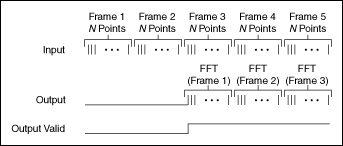### Timing Diagram Inside the Single-Cycle Timed Loop When Throughput is Equal to 1 cycle / input

The following diagram demonstrates timing when you use this Express VI inside a single-cycle Timed Loop with Throughput equal to 1 cycle / input.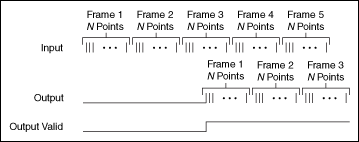### Timing Diagram Inside the Single-Cycle Timed Loop When Throughput is Not Equal to 1 cycle / input

The following diagram demonstrates timing when you use this Express VI inside a single-cycle Timed Loop with Throughput not equal to 1 cycle / input.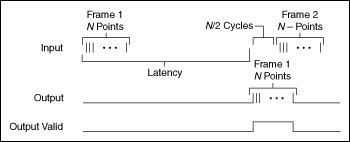### Avoiding Data Loss During FFT Calculation

In a single-cycle Timed Loop, this Express VI does not accept or return values while computing the FFT. During this time, if the system or another node sends data to this Express VI, LabVIEW discards the data. This might happen if this Express VI receives data as part of a complicated or non-uniform pattern.

To ensure no data is lost, create a FIFO to hold data until this Express VI accepts values again. Ensure that the FIFO you create is large enough to hold all data points that collect during the FFT calculation time. To roughly estimate the size of the FIFO you need to create, divide the latency of this Express VI by the average system throughput.

### Selecting the Appropriate Input/Output Index Pattern (Single Channel, Multiple Samples Input Format)

When using the Single Channel, Multiple Samples input format, you must select the appropriate Input/Output Index Pattern. If the data source of an FFT Express VI is the FPGA I/O node, select Continuous input indexes / M-interval output indexes. If the data source is the output of another FFT Express VI, such as when performing inverse FFT on FFT results, ensure that the input pattern of the second FFT Express VI is the same as the output pattern of the first FFT Express VI. Otherwise, the FFT results will be inaccurate.

For a 4096-point FFT with 4 samples per input using the Continuous input indexes / M-interval output indexes Input/Output Index Pattern (where M equals 1024), the indexes of the input samples are 0, 1, 2, 3 in the first valid input cycle, 4, 5, 6, 7 in the second cycle, and so on. The indexes of the output samples are 0, 1024, 2048, 3072 in the first valid output cycle, 1, 1025, 2049, 3073 in the second cycle, and so on. The following figure shows a graphical representation of such a Continuous input indexes / M-interval output indexes Input/Output Index Pattern: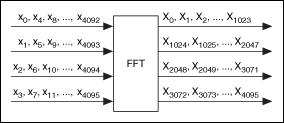Conversely, if you select the M-interval input indexes / Continuous output indexes Input/Output Index Pattern, the output will be in the continuous pattern. Compared with the previous example, the patterns of input and output are reversed. The following figure shows a graphical representation of such an M-interval input indexes / Continuous output indexes Input/Output Index Pattern: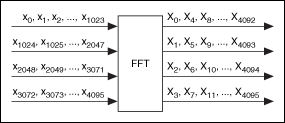### Implementation Goal Considerations (Single Channel, Multiple Samples Input Format)

Theoretically, performing an N-point FFT on fixed-point input data results in output data with word lengths increased by log2(N)+1 bits compared to the input data. When you select Accuracy in the Implementation Goal section of the configuration dialog box, the FFT Express VI extends the word length of the input data to the output word length by padding zeros, and all internal complex multiply operations use this word length. Conversely, when you select Resource usage, the FFT Express VI increases the word length stage-by-stage from input to output. Therefore, the bit width of complex multipliers also increases stage-by-stage.

When you select Resource usage, the complex multipliers retain fewer bits than when you select Accuracy, so the increased log2(N)+1 bits in the output data are not as accurate compared to when you select Accuracy. Selecting Resource usage saves FPGA resources because the bit widths of the complex multipliers and internal registers are smaller than the bit widths that result when you select Accuracy. You potentially can achieve a higher FPGA clock rate at compilation when you select Resource usage because of the resulting shorter word lengths used for complex multipliers.

## Examples

Refer to the following VIs for examples of using the FFT Express VI:

• labview\examples\CompactRIO\FPGA Fundamentals\FPGA Math and Analysis\FFT\FFT.lvproj
• labview\examples\R Series\FPGA Fundamentals\FPGA Math and Analysis\FFT (1 Channel, N Samples)\FFT (1 Channel, N Samples).lvproj
• labview\examples\R Series\FPGA Fundamentals\FPGA Math and Analysis\Cross-correlation Using FFT (1 Channel, N Samples)\Cross-correlation Using FFT (1 Channel, N Samples).lvproj
• labview\examples\R Series\FPGA Fundamentals\FPGA Math and Analysis\FFT\FFT.lvproj